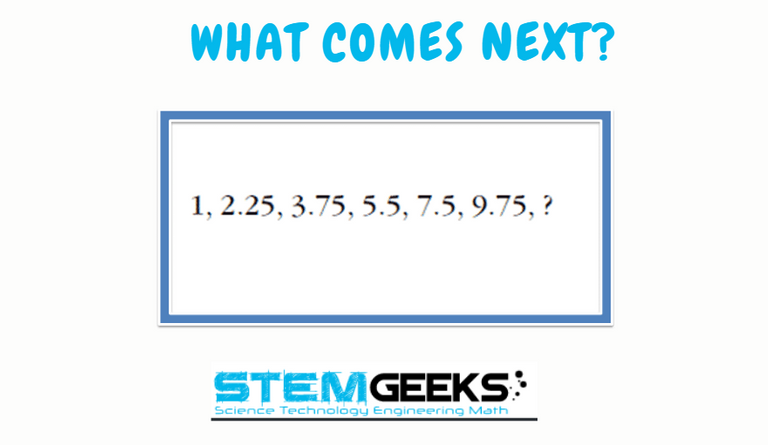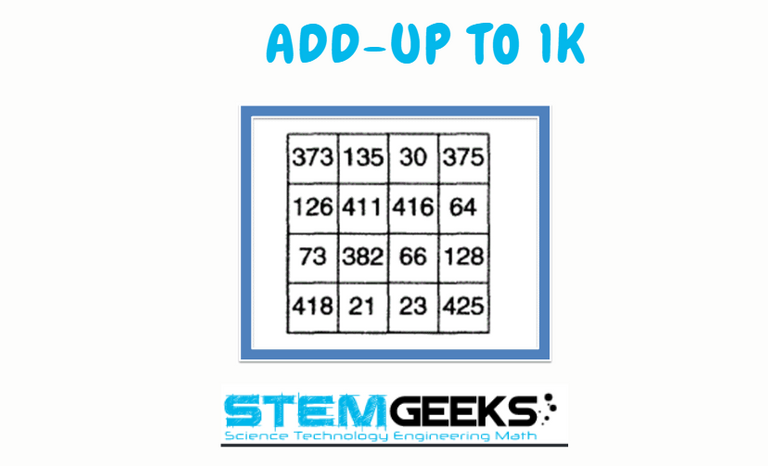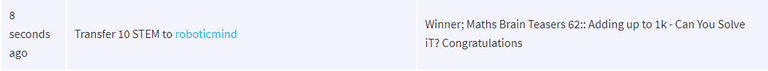# Maths Brain Teasers 63:: Can You Complete The Sequence???

in STEMGeekslast month

Hey All; @StemGeeks Mathematician;

Without further, ado, let's get to our puzzle for today:: Maths Brain Teasers 63:: Can You Complete The Sequence???Have a close look at the image given and see if you find out the right sequence to replace the question mark with the right Number. Find the hidden pattern to complete the puzzle. There is logic to resolve the puzzle; try finding out the logic and it should be resolved in seconds.. Guesswork isn't going to help...

With that, I'll leave you all with the Maths Brain Teasers 63:: Can You Complete The Sequence??? Good luck solving iT...

##### Maths Brain Teasers 62:: Adding up to 1k - Can You Solve iT?- Solved with ExplanationLet's have a look at the Step by Step instructions to Solve the Maths Brain Teasers 62:: Adding up to 1k - Can You Solve iT?

We had a script created in Python by @roboticmind to solve the puzzle.. What better than a machine finding the answer for you.. Just input the numbers and it will do all the calculations for You...

Hence the Answer to the Maths Brain Teasers 62 is ::

### STEM token GiveAway

I'll be again doing a giveaway of STEM tokens to the lucky random winner with the correct answer. For the last contest, which was Maths Brain Teasers 62:: Adding up to 1k - Can You Solve iT?.

We had 3 entries and I happy to see the engagement by everyone. At the same time, it's great to see the detailed answers provided by everyone as to how they arrived at the solution of the puzzle.. Well done guys.. Way to GO... Now the results part; I'm going to use a random picker tool to select a Winner. And the Winner is....Congratulations @roboticmind; You WIN yourself 10 STEM tokens. You should be having the rewards in your STEM Wallet Soon.

### Math Quote for the Day::

Here is the motivation to solve the Maths Equation Puzzle?If you like my work, then please spread the Word.. that we do have the Math Brain Teasers competition here @StemGeeks platform. Reblog is much appreciated.

Best RegardsFind Me on the Other Social Media Platforms::PS:: All the Maths Brain Teasers; are made by me using the Pro Canva License Version

Sort:

12.25.

Increment by 1.25 for the first time then increase the increment by 0.25 for each addition increment. 1+1.25+1.5+... would give the last increment as 2.5. Thus the answer is 9.75+2.5=12.25.

It's been a while since i participated.

1 + 1.25 = 2.25
2.25 + 1.50 = 3.75
3.75 + 1.75 = 5.5
5.5 + 2 = 7.5
7.5 + 2.25 = 9.75
9.75 + 2.50 = 12.25

2.25 - 1 = 1.25
3.75 -2.25 = 1.5
5.5 - 3.75 = 1.75
7.5 - 5.5 = 2
9.75 -7.5 = 2.25
x - 9.75 = ?
Looking at the pattern, we see that each time we subtract 2 consecutive numbers (going from the smallest to largest) the difference increase by 0.25
So x - 9.75 = 2.5
We solve for x and get: x = 2.5 + 9.75 = 12.25
Therefore the number that replaces the question mark is 12.25